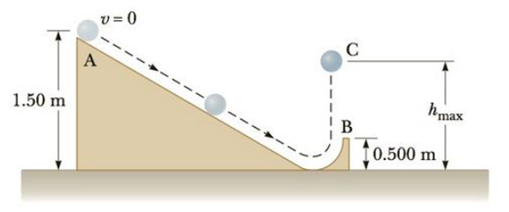Chapter 8, Problem 59P

Chapter
Section
Textbook Problem

A 2.00-kg solid, uniform ball of radius 0.100 m is released from rest at point A in Figure P8.59, its center of gravity a distance of 1.50 m above the ground. The ball rolls without slipping to the bottom of an incline and back up to point B where it is launched vertically into the air. The ball rises to its maximum height hmax at point C. At point B, find the ball’s (a) translational speed and (b) rotational speed ωB. At point C, find the ball’s (c) rotational speed ωC and (d) maximum height of its center of gravity.Figure P8.59

(a)

To determine
The translational speed vB .

Explanation

Given info: The mass of the ball is 2.00 kg. The distance of center of gravity from the ground is 1.50 m.

From Conservation of energy

mgyA=12mvB2+12IωB2+mgyB

• I is the moment of inertia of the ball.
• ωB is the rotational speed.
• m is the mass of the ball.
• g is the acceleration due to gravity.
• yA is the height of point A from the ground.
• yB is the height of point B from the ground.

The moment of inertia of the ball is,

I=25mr2

The rotational speed is,

ωB=vBr

From the above equations,

mgyA=12mvB2+15mvB2

(b)

To determine
The rotational speed ωB .

(c)

To determine
The rotational speed at the point C ωC .

(d)

To determine
The maximum height of the center of gravity.

Still sussing out bartleby?

Check out a sample textbook solution.

See a sample solution

The Solution to Your Study Problems

Bartleby provides explanations to thousands of textbook problems written by our experts, many with advanced degrees!

Get Started

Find more solutions based on key concepts